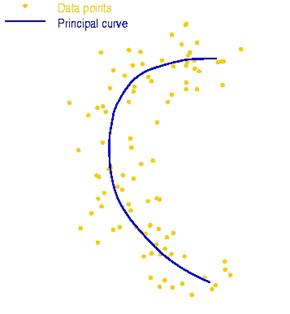# PRML读书笔记——连续潜在变量

## 主成分分析

PCA可以被定义为数据在低维线性空间上的正交投影，这个线性空间被称为主⼦空间，使得投影数据的⽅差被最⼤化。下面从两个角度给出定义

### 最大方差形式

1Nn=1N{uT1xnuT1x}2=uT1Su1

S=1Nn=1N(xnx)(xnx)T

L(u1,λ1)=uT1Su1+λ1(1uT1u1)

u1$u_{1}$求导，得到：

Su1=λ1u1

### 最小误差形式

uTiuj=δij

xn=i=1Dαniui

xn=i=1D(xTnui)ui

x˜n=i=1Mzniui+i=M+1Dbiui

J=1Nn=1Nxnx˜n2

znj=xTnuj

bj=xTuj

J=1Nn=1Ni=M+1D(xTnuixTui)2=i=M+1DuTiSui

## 概率PCA

### 基本概念

⾸先显式地引⼊潜在变量z$z$，对应于主成分⼦空间。接下来我们定义潜在变量上的⼀个⾼斯先验分布p(z)$p(z)$以及以潜在变量的值为条件，观测变量x$x$的⾼斯条件概率分布p(x|z)$p(x | z)$。具体来说，z$z$上的先验概率分布是⼀个零均值单位协⽅差的⾼斯分布

p(z)=N(z|0,I)

p(x|z)=N(x|Wz+u,σ2I)

x=Wz+u+ϵ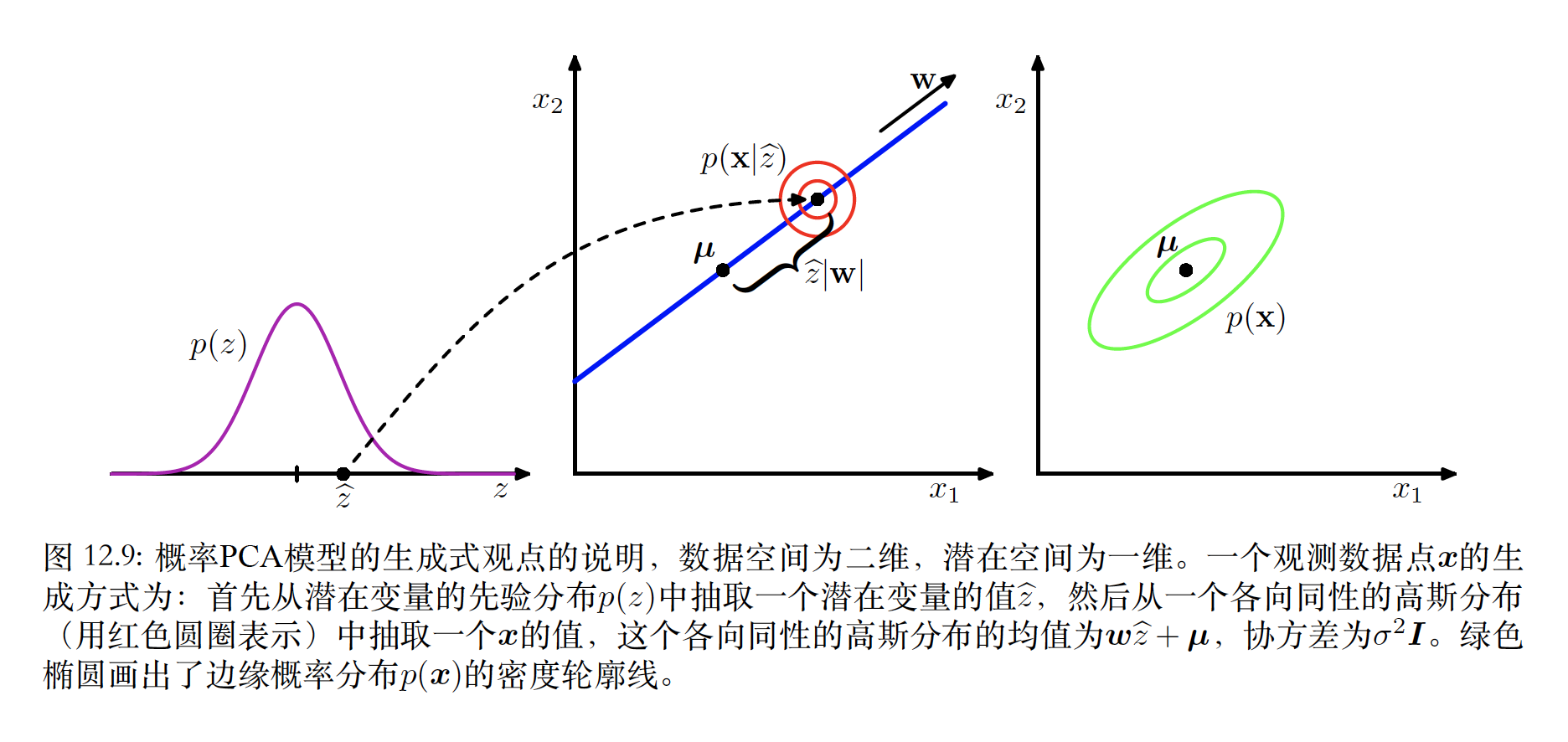### 最大似然求解

p(x)=p(x|z)p(z)dz=N(x|u,C)

C=WWT+σ2I

ln p(X|u,W,σ2)=n=1Nln p(xn|W,u,σ2)=ND2ln(2π)N2lnC12n=1N(xnu)TC1(xnu)

u=x

WML=UM(LMσ2I)12R

σ2$\sigma^{2}$求导，得到：

σ2ML=1DMi=M+1Dλi

### 贝叶斯PCA

p(W|α)=i=1M(αi2π)D2exp{12αiwTiwi}

p(X|α,u,σ2)=p(X|W,u,σ2)p(W|α)dW

αnew=DwTiwi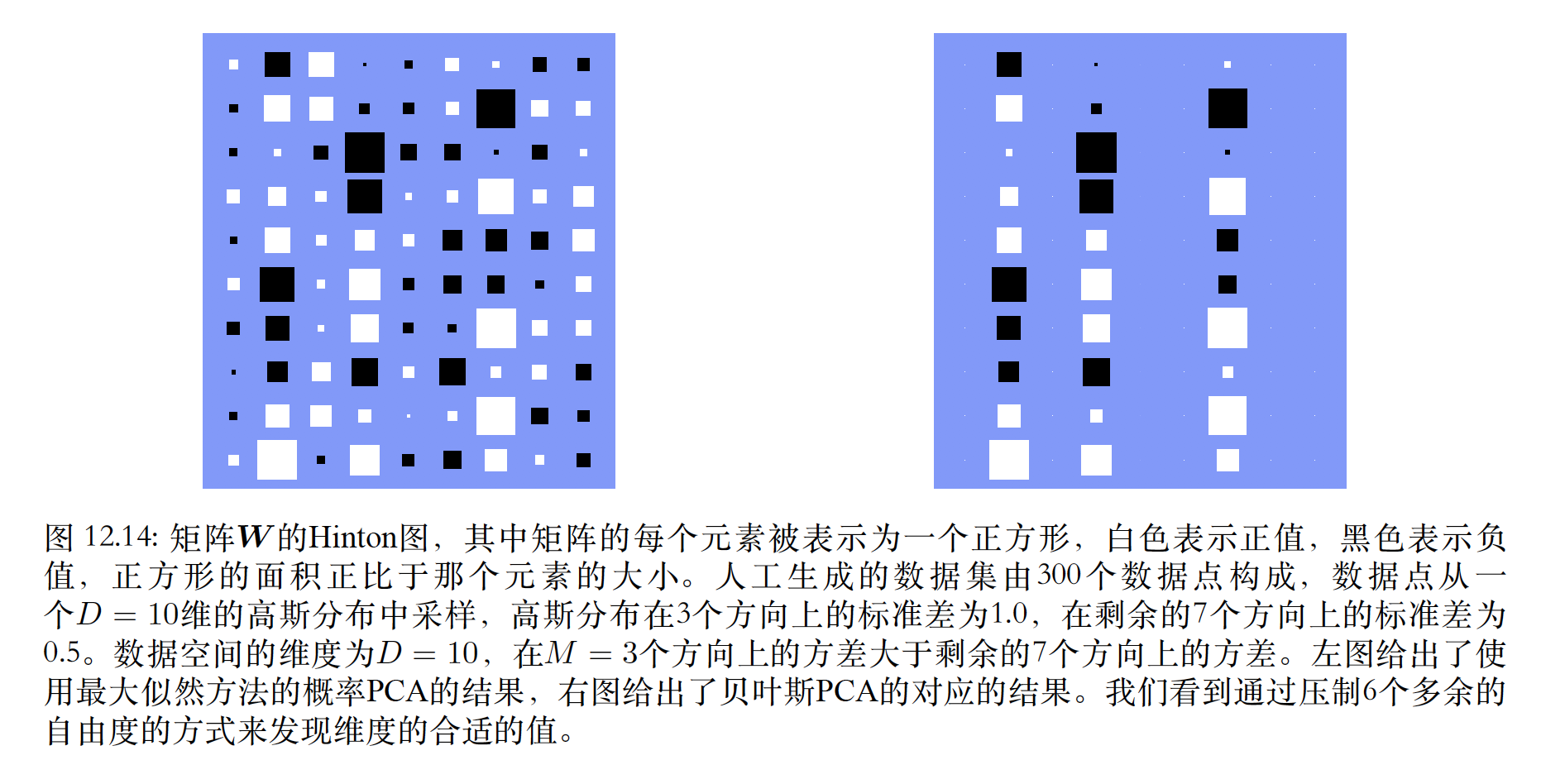## 核PCA

Sui=λiui

S=1Nn=1NxnxTn

C=1Nn=1Nϕ(xn)ϕ(xn)T

Cvi=λivi

1Nn=1Nϕ(xn){ϕ(xn)Tvi}=λivi

vi=n=1Nainϕ(xn)

1Nn=1Nϕ(xn)ϕ(xn)Tm=1Naimϕ(xm)=λin=1Nainϕ(xn)

1Nn=1Nk(xl,xn)m=1Naimk(xn,xm)=λin=1Naink(xl,xn)

Kai=λiNai

1=vTivi=n=1Nm=1Nainaimϕ(xn)Tϕ(xm)=aTiKai=λiNaTiai

yi(x)=ϕ(x)Tvi=n=1Nainϕ(x)Tϕ(xn)=n=1Naink(x,xn)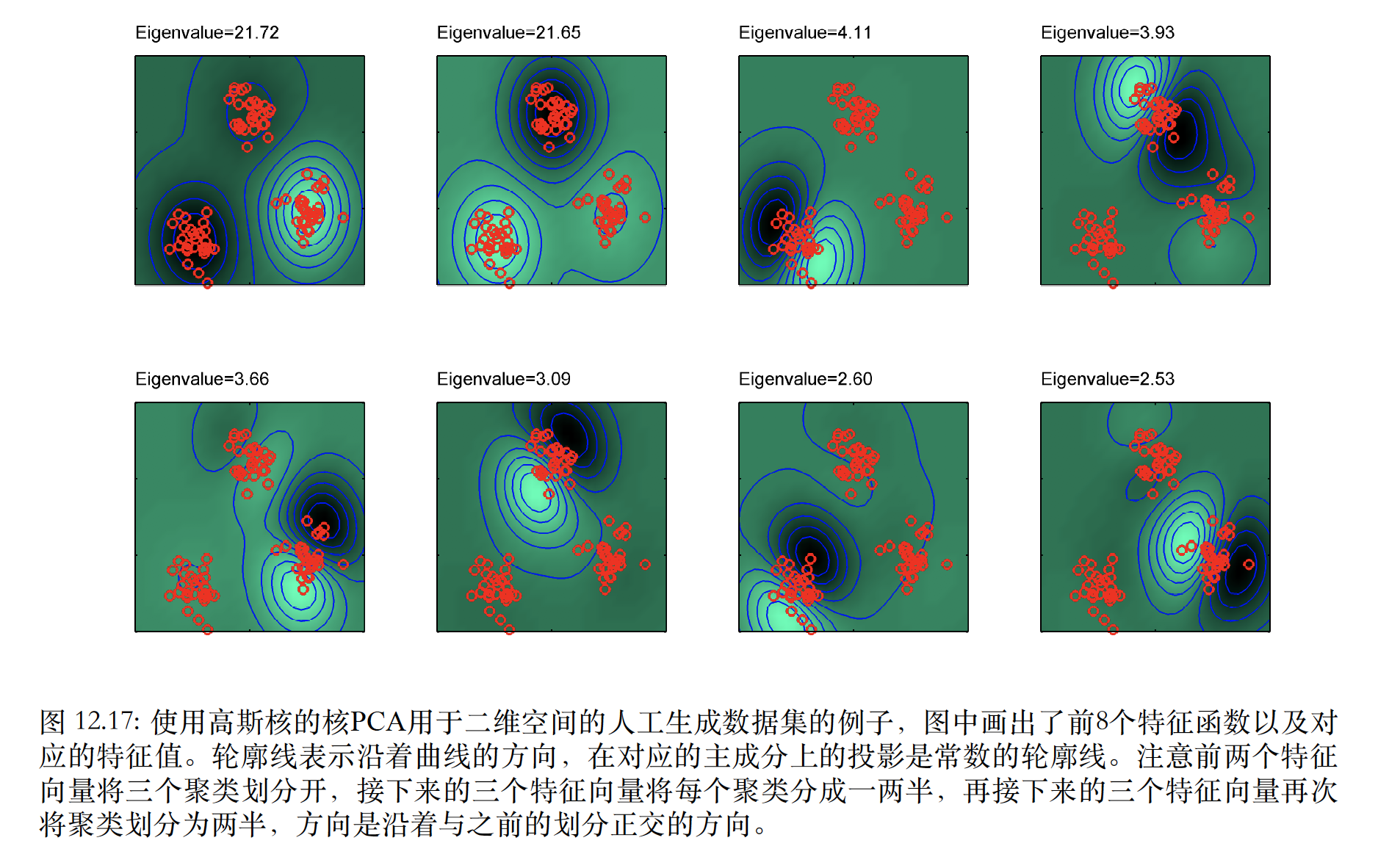## 非线性隐含变量模型

p(z)=j=1Mp(zj)

### 自关联网络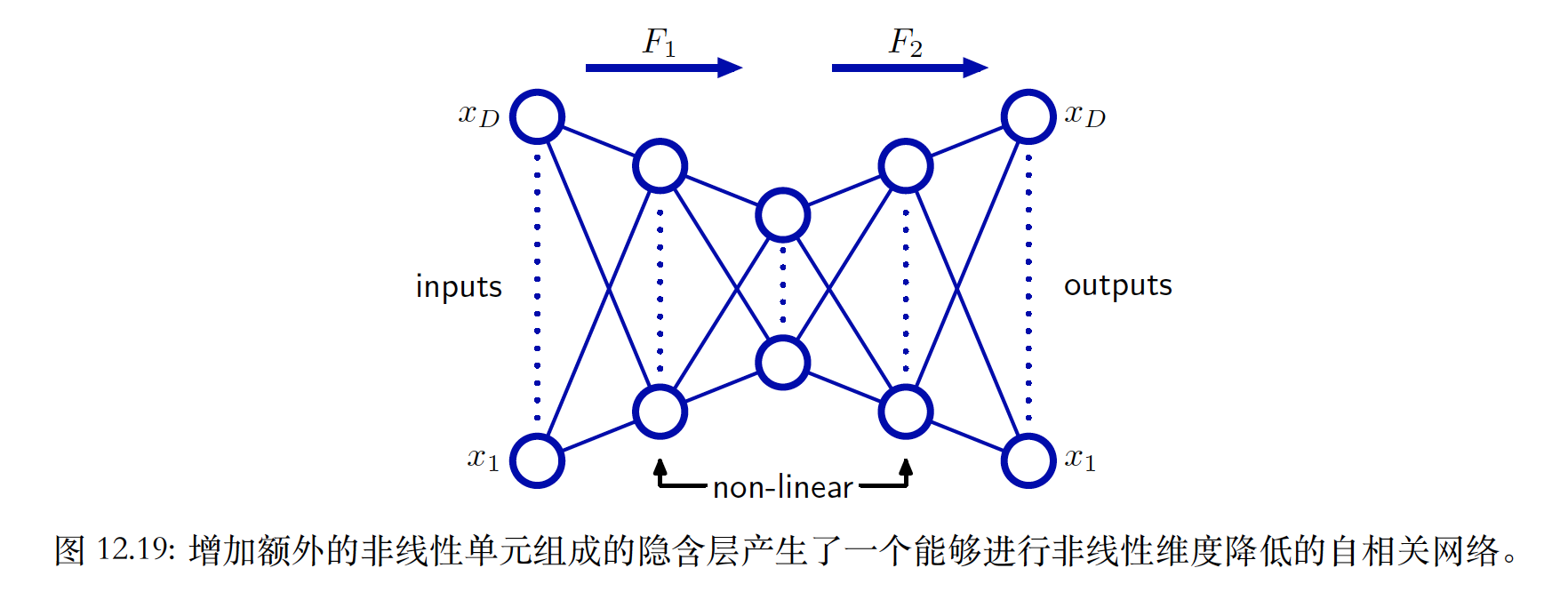### 对非线性流形建模